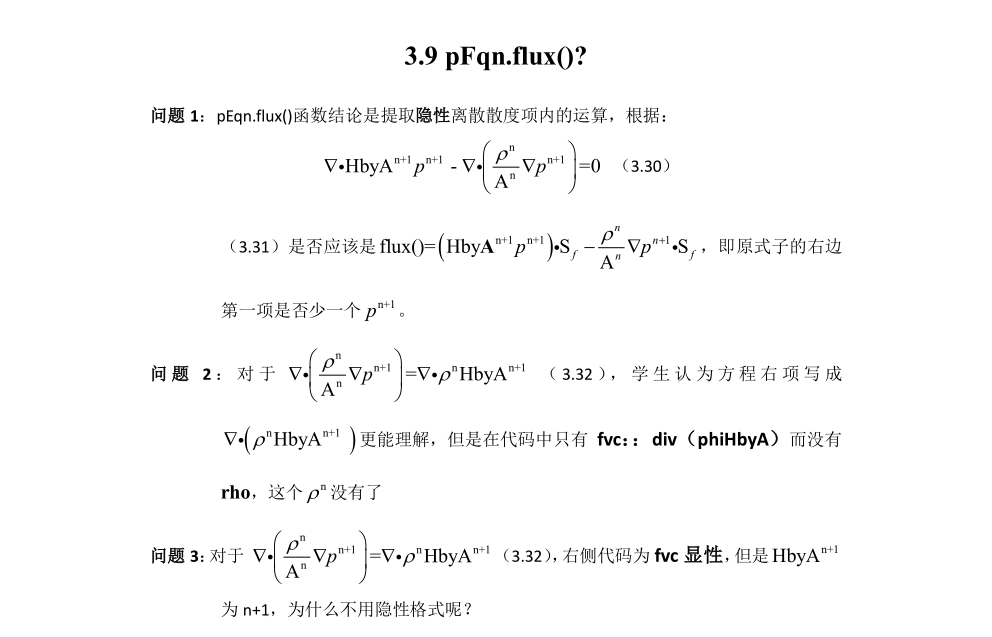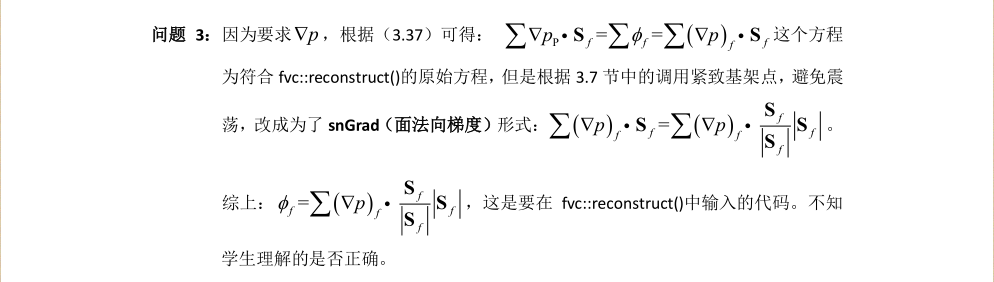# 《无痛苦N-S方程笔记》求指导

•老师，学生理解力比较差，好几个地方不懂，希望老师有时间的时候能够指点一二，非常感谢！
@东岳

• 现在单说$\sum\bfU_P\cdot\bfS_f=\sum\bfU_f\cdot\bfS_f$，这里$\bfU_P$是定义在网格体心的，因此只能这么写：
$$\bfU_P\cdot\sum\bfS_f=\sum\bfU_f\cdot\bfS_f$$
有点区别，最后一个方程类似于做平均。另外，$\bfU_f\cdot\bfS_f$输入的不是$\bfU_f$而是作为通量$\phi_f$进行输入。因此是通过通量$\phi_f$获得$\bfU_P$。有点像是：
$$\frac{1a+2b+3c}{a+b+c}(a+b+c)=(1a+2b+3c)$$
很明显前面$\frac{1a+2b+3c}{a+b+c}\neq 1 \neq 2 \neq 3$，同时$\frac{1a+2b+3c}{a+b+c}$像是一种平均操作，即reconstruct

谢谢！我简单改了一下，周末再系统看一下。

• @东岳 谢谢老师，这个问题当时思维定式了，把U_p提出来，立马就懂了。

CFD中文网 2016 - 2020 | 京ICP备15017992号-2